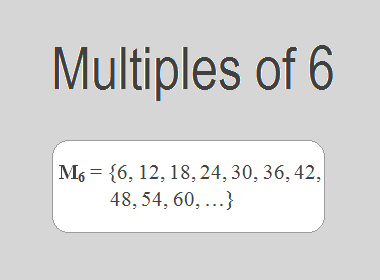# Multiples of 6The Multiples of 6 are 6, 12, 18, 24, 30, 36, 42, 48, 54, 60, … i.e. M6 = {6, 12, 18, 24, 30, 36, 42, 48, 54, 60, …}. Multiples of 6 are the series of numbers obtained by multiplying 6 with the natural numbers.

6 × 1 = 6

6 × 2 = 12

6 × 3 = 18

6 × 4 = 24

6 × 5 = 30

6 × 6 = 36

6 × 7 = 42

6 × 8 = 48

6 × 9 = 54

6 × 10 = 60

And so on.

From this series, we observe that the multiples of 6 can be derived by multiplying 6 by natural numbers in ascending order. These multiples form an arithmetic sequence with a common difference of 6.

## Properties and Patterns of Multiples of 6

Multiples of 6 possess several interesting properties that make them unique. Here are a few notable ones:

1.    Divisibility by 6: Every multiple of 6 is divisible by 6 without leaving a remainder. This property stems from the fact that when we divide any multiple of 6 by 6, the quotient is always an integer.

2.    Divisibility by 2 and 3: Since 6 is divisible by both 2 and 3, all multiples of 6 are also divisible by both 2 and 3. Divisibility by 2 implies that all multiples of 6 are even numbers, while divisibility by 3 suggests that the sum of the digits of any multiple of 6 is also divisible by 3.

3.    Repeating Pattern of Digits: When we examine the units digit of multiples of 6, a fascinating pattern emerges. The units digit repeats in a cycle of 6, 2, 8, 4, 0, and again 6, as we proceed to higher multiples. For instance, the units digit of 6 is 6, the units digit of 12 is 2, and so on.

********************

********************

## Applications of Multiples of 6

The study of multiples of 6 finds applications in various fields, including mathematics, science, and everyday life:

1.    LCM: One of the applications of multiples of 6 is in finding the lowest common multiple (LCM) of two or more numbers. The LCM is the lowest multiple that two or more numbers have in common. For example, to find the LCM of 6 and 4, we need to find the multiples of both numbers and identify the lowest multiple they have in common. The multiples of 6 are 6, 12, 18, 24, 30, 36, 42, 48, 54, 60, … etc. The multiples of 4 are 4, 8, 12, 16, 20, 24, 28, 32, 36, 40 … etc. The lowest multiple that they have in common is 12. Therefore, the LCM of 6 and 4 is 12.

2.    Timekeeping: The division of an hour into 60 minutes and each minute into 60 seconds is based on the concept of multiples. By dividing 60 by 6, we obtain 10, which corresponds to the number of minutes in one-tenth of an hour.

3.    Music: In music theory, multiples of 6 play a crucial role in understanding rhythm. The time signatures and beats per measure are based on multiples of 6, allowing musicians to create harmonious compositions.

## Conclusion:

The Multiples of 6 are the numbers obtained by multiplying 6 with natural numbers. The multiples of 6 are 6, 12, 18, 24, 30, 36, 42, 48, 54, 60, … etc. The multiples of 6 have various properties, such as divisibility by 6, divisibility by 2 and 3, repeating pattern of digits, etc. The multiples of 6 have several applications in mathematics, such as finding the LCM, timekeeping, and music.